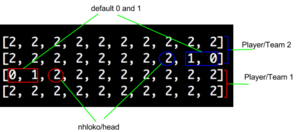In improve this we take a look at a reader submitted test, user interface, story or block of code and we try and improve it, without context, explaining what we did as we went.

This month’s Improve This comes from Musa. Musa started with some code that he wrote before joining ThoughtWorks. He wanted to look back at what he’d learnt and see how the code he used to write has changed.

The code below populates sets up the initial board state for the game of Ncuva.

`a = [[], [], [], []]width = 10length = 4stones = 2 # or 3`
`for i in range(length):	for j in range(width):		a[i].append(stones)		if i == 1 and j == width - 2:  		a[i][j] = 1		if i == 1 and j == width - 1:  		a[i][j] = 0		if i == 2 and j == 0:  		a[i][j] = 0		if i == 2 and j == 1:  		a[i][j] = 1		if stones == 3:  		if i == 1 and j == width - 3:  			a[i][j] = 2  		if i == 2 and j == 2:  			a[i][j] = 2`

The for loop makes use of 3 global variables: the length, width and number of stones in each slot. The length and width control how much looping is done. The two cases for the number of stones catered for here are 2 and 3.

Executing this code will give rise to a structure like this:As you can see from the figure displayed above, there is a pattern at work here. The pattern screams out: there are too many 2’s! We gather that we do not have to loop through all of a to know how many stones to place in each slot.

On having noticed the pattern, we go ahead and fill a with 2 or 3 stones according to the user’s choice. How many corrections would we have to do if we filled a with 2’s? Would this approach provide any savings? 6 (at best 4) corrections are better than 4x10x5 (at worst 4x10x7) checks.

For the time being we leave out all the if statements.

`def array(stones, length):   return [stones]*length`
`a = map(array, ([tincuva]*width, length))`

Instead of going another iteration around the board, we just pick the specific indices that have been assigned wrongly and give them correct values.

In fact, this for-loop can be optimised out by using the built-in map function.

According to ncuva rules, at the beginning of each game, the first and second slots in the second and third rows will always have no stone and 1 stone, respectively. If you look at the figure, you should notice that these slots are mirror images of each other. So we go ahead and assign these slots accordingly.

`a[width - 2] = 1a[width - 1] = 0a = 0a = 1if stones == 3:	a[width - 3] = 2	a = 2`

Since Python has the ability to count from the end of a list going backwards, we can replace the calculation width – some_number with the negative of some_number. In fact, we do not need the if as a will not be changed incorrectly for stones = 2 or stones = 3.

`a[-3] = 2a[-2] = 1a[-1] = 0a = 0a = 1a = 2`

We now introduce a method so that a can be populated whenever needed and not just once when the game starts. set_board_up takes length, width and stones as input. This means we can get rid of these three lines:

`width = 10length = 4stones = 2 # or 3`

`board = [[], [], [], []]`
`def set_board_up(length, breadth, tincuva):  board = map(array, ([tincuva]*breadth, length))  board[-3] = 2  board[-2] = 1  board[-1] = 0  board = 0  board = 1  board = 2  return board`
`board[-1], board = 0, 0board[-2], board = 1, 1board[-3], board = 2, 2`